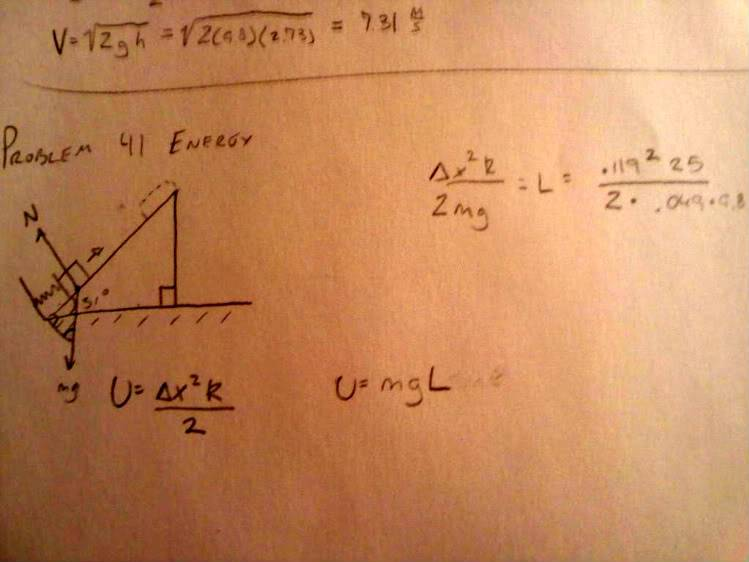# Ice pressed against a spring

## Homework Statement

A 49 g ice cube can slide without friction up and down a 31° slope. The ice cube is pressed against a spring at the bottom of the slope, compressing the spring 11.9 cm. The spring constant is 25 N/m. When the ice cube is released, what distance will it travel up the slope before reversing direction?

Pi=Pf
U=mgx, kx^2/2
K=mv^2/2

## The Attempt at a Solution

Initially U=k*x^2/2
then at the end U=mgLsin31
so then i combined the 2 equations and solve for L and got L=(k*x^2)/(2*.049*9.8*sin(31))
it is saying its wrong....what am i doing wrong?

Doc Al
Mentor
Looks OK to me, assuming the distance is measured from the compressed position.

Yeah that is right if you are considering from compressed pos. If it is from relaxed position, you might have to subtract 0.119. Is the answer 0.597m?

yeah thas my anwser but its wrong.

I tried both .7157 and when you subtract .597 and they both are wrong.

Last edited:
bump..... I still dont know what I am doing wrong

I have one try left!?! FML

here is a copy of my work....Ui=(delta x^2)*k/2
Uf=mgL

solved for L= (delta x^2)*k/2mg

Doc Al
Mentor
As I said earlier, there's nothing wrong with your work. But online systems can be flaky. Since they gave the compression in units of cm, perhaps that's the units they want.

Did you post the entire problem word for word exactly as it was given?

Doc Al
Mentor
Ui=(delta x^2)*k/2
Uf=mgL

solved for L= (delta x^2)*k/2mg
For some reason you chose to find the vertical distance instead of the distance up the slope. I'd say your first post was correct.

o ok I am almost positive here ill copy and paste it again......

A 49 g ice cube can slide without friction up and down a 31° slope. The ice cube is pressed against a spring at the bottom of the slope, compressing the spring 11.9 cm. The spring constant is 25 N/m. When the ice cube is released, what distance will it travel up the slope before reversing direction?

tiny-tim
Homework Helper
A 49 g ice cube can slide without friction up and down a 31° slope. The ice cube is pressed against a spring at the bottom of the slope, compressing the spring 11.9 cm. The spring constant is 25 N/m. When the ice cube is released, what distance will it travel up the slope before reversing direction?

Initially U=k*x^2/2
then at the end U=mgLsin31
so then i combined the 2 equations and solve for L and got L=(k*x^2)/(2*.049*9.8*sin(31))
it is saying its wrong....what am i doing wrong?

Hi talaroue!(try using the X2 tag just above the Reply box)

You need sin31º times (x + .119),

and (assuming the ice stays on the spring) you need kx2 - k(.119)2he didnt have his C++ language right, aka he forgot to incorporate the degrees into the anweser. Thanks eveyrone